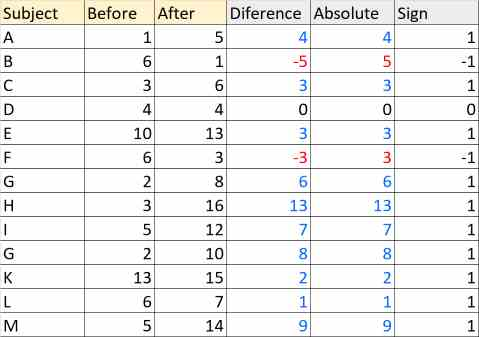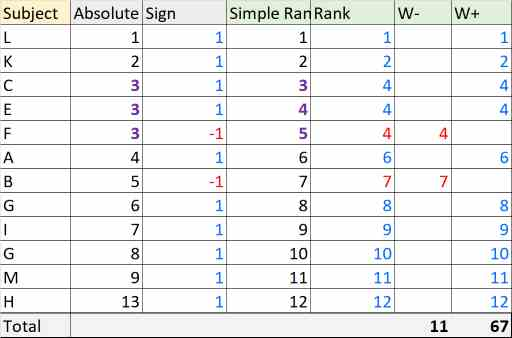# Wilcoxon Signed-Rank test (Go To The Calculator)

The Wilcoxon Signed-Rank test is a nonparametric test, it checks continuous or ordinal data for a significant difference between two dependent groups. The test finds the deltas of the two groups. Then, it sorts the pairs by the absolute value of the deltas.

## When to use?

The test usually comes as a failsafe option to a paired t-test when the test doesn't meet the normality assumption, or contains many outliers.
A paired t-test compares the means of the two groups, while a Wilcoxon Signed-Rank test compares the entire distributions. If the two groups have a similar distribution curve, the test will also check if the median of the deltas is the expected value.
A paired t-test is slightly stronger than a Wilcoxon Signed-Rank Test. A Wilcoxon Signed-Rank Test has 95% efficiency in comparison to a paired t-test. If the population is similar to a normal distribution and reasonably symmetric, it is better to use a pair t-test.

• Not Normal, the data is not normally distributes
• Ordinal data, but not interval scaled. You know the order but not the differences between the values. for example: Unhappy, Neutral, Happy
• Outliers the test is more robust to outliers than t-test

## Assumptions

• Dependent pairs, but independence between the pairs
• Ordinal / Continuous the compared data consist of ordinal data or continuous data
• Shape the data is not necessarily normally distributed but should have a similar shape. If not you can compare the ranks but not the median of the differences

## Calculate W

• Calculate the difference between the pairs, for example after treatment value minus before treatment value
• Exclude pairs with zero difference and sort by the absolute rank
• Sort the data from low absolute value to high absolute value
• Rank the list, the lower absolute value get rank of 1 , the second rank of 2, etc
When having ties group, identical value for several observations the rank will be the average of the ranks for the entire group
Give each rank a sign positive if the difference is positive and negative if the difference is negative.
• Accumulate the ranks
Ri - the absolute rank of the i rank difference.
n - number of pairs, where the difference is not zero. $$W_+=\sum_{i=1}^{n}{R_{i}}\enspace where \enspace sign\enspace is\enspace positive$$ $$W_-=\sum_{i=1}^{n}{R_{i}}\enspace where \enspace sign\enspace is\enspace negative$$ $$W_++W_-= \frac{n(1+n)}{2} \enspace sum \enspace of \enspace arithmetic \enspace progression$$ Since the distribution is symmetrical, usually w is the minimum between w+ and W-. $$W=min(W_+ , W_-)$$ It is good for two tails test, but for one tail the test will always assume that H1 is the sample with bigger values is bigger than the sample with the smaller values. In this website we chose: statistics=W-, in this way we can calculate left tail or right tail like any other test.

• Check the critical W in the table, reject H0 if W < Wcritical.

## Critical Value

When n is small, the tool will use the exact value from tables, the exact critical value is accurate (for common significant levels ), while the p-value is usually interpolated from two values in the table. The tool uses log interpolation which is more accurate with small p-values and less accurate with bigger p-values. (the common significant levels are small: 0.05, 0.01).
There is no consensus about what is a small n. When Method="automatic" the tool uses the Exact method when n ≤ 40, and normal approximation when n > 40.
When Method="z approximation" the tool uses only the normal approximation.

### Statistical tables

Calculated the critical W from a statistic table.

### Corrected normal approximation

To get more accurate results, the tool uses continuity correction and ties correction. ties is a group of observations with the same value. $$z = \frac { W_- - \mu_w + C_{continuity}} {\sigma_w}\\ \mu= \frac {n(n+1)} {4}\\ \sigma^2= \frac {n(n+1)(2n+1)} {24} - C_{ties}$$

#### Continuity correction

When using Continuous distribution for a discrete data. it is better to use the continuity correction.
If  W > μ , Ccontinuity = - 0.5
If  W < μ , Ccontinuity = 0.5
When using a continuous data, Ccontinuity = 0.

#### Ties correction

$$C_{ties} = \sum_{i=1}^{t}{\frac{f_t^3-f_t}{48}}$$ t - group number of ties
f_t - number of values in group t

example: [1,2,2,2,3,4,5,5,5,5,6,7,8,8,8,8,8,8,9,10]
$$group_1: [2,2,2], \quad f_1=3\\ group_2: [5,5,5,5], \quad f_2=4\\ group_3: [8,8,8,8,8,8], \quad f_3=6$$

## Example

In the following example, we check the number of questions answered correctly by the same subject, before and after performing a training.
The significant level (α) is 0.05;

• Calculate Difference Absolute and Sign: (Difference = After- Before, Absolute = Absolute(Difference), Sign = sign(Difference) )• The sample size contains 13 pairs. Exclude D pair with zero difference
n = 13 - 1 = 12
• Sort by Absolute value• Simple Rank - rank by the Absolute value, the lower Absolute value get 1 rank, the second 2 etc.
• Rank - usually will be the same as Simple Rank. subjects C,E,F have identical Absolute value: 3. The rank is the average of the simple rank values
For subjects C,E,F
$$\frac{3+4+5}{3}=4$$ W- = 4 + 7 = 11
W+ = 1+ 2 + 4 + 4 + 6 + 8 + 9 + 10 + 11 + 12 = 67 W = min(11 , 67) = 11

### Statistical tables

#### Two tailed (H0: Before = After)

• Critical Value
Check the the two tails statistic table, for α = 0.05, n = 12.
Critical W is 13.
• P-value
• For α=0.02, critical W is 9.
For α=0.05, critical W is 13.
Since 11 is between 9 and 13, the p-value will be between 0.02 and 0.05.
The tool will do a logarithmic extrapolation: p-value = 0.032
• Decision
Since p-value < α (0.032 < 0.05 ) or alternatively since W < Wcritical (11 < 17) we reject the H0
• Website
The website uses W- instead of W.
Left critical W- = 13.
Right critical W- = n (1 + n) / 2 - Left critical W = 12 * 13 / 2 - 13 = 65.
Since W- (11) is in the following range: [13,65], accept H0. When W- = 13 or 65 you still accept the H0.

#### Left tail (H0: Before ≥ After)

• Critical Value
Check the the two tails statistic table, for α = 2 * 0.05 = 0.1, n = 12.
The critical W is 17.
• P-value
P-value = p-value(Two tailed) / 2 = 0.032 / 2 = 0.016
• Decision
Since p-value < α (0.016 < 0.05) or alternatively since W- < Wcritical(11 < 17 ) reject H0.

#### Right tail (H0: Before < After)

• Critical Value
Check the the two tails statistic table, for α = 2 * 0.05 = 0.1, n = 12.
The in the table is is 17.
The critical W is n(1 + n) /2 - value from the table = 12 * 13 / 2 - 17 = 61.
• P-value
P-value =1 - p-value(Two tailed) / 2 = 1 - 0.016 = 0.984
• Decision
Since p-value < α (0.984 < 0.05) or alternatively since W- < Wcritical (11 < 61), accept H0

### Corrected normal approximation

• There is only one tie group (t=1), that contains 3 values: f1=3
$$C_{ties} = \sum_{i=1}^{1}{\frac{3^3-3}{48}}=0.5$$
• Since W<μ , Ccontinuity=0.5 $$\mu_w= \frac {n(n+1)}{4}=\frac {12(12+1)}{4}=39$$ $$\sigma^2_w= \frac {n(n+1)(2n+1)} {24} - C_{ties}= \frac {12(12+1)(2*12+1)} {24} - 0.5=162.5 \quad => \sigma_w=12.7279$$ $$Z = \frac { W_- - \mu_w + C_{continuity}} {\sigma_w} = \frac { 11 - 39 + 0.5} {12.7279}=-2.16$$
• P(z≤Z) = P(z≤-2.16) = 0.01539.

#### Two tailed (H0: Before = After)

• p-value = 2 * 0.01539 = 0.03078.
• Since 0.03078 < 0.05, reject H0.

#### Left tail (H0: Before ≥ After)

• p-value = P(z≤-2.16) = 0.01539.
• Since 0.01539 < 0.05, reject H0.

#### Right tail (H0: Before < After)

• p-value =1 - P( z≤ -2.16) = 1 - 0.01539 = 0.98461.
• Since 0.98461 > 0.05, accept H0.